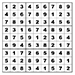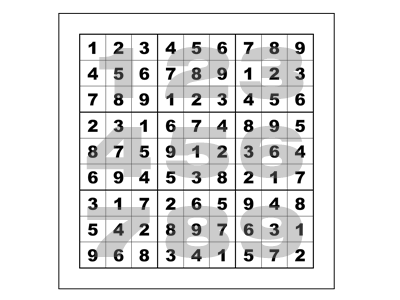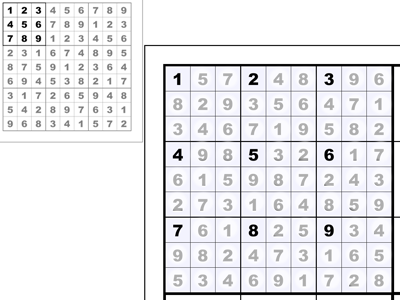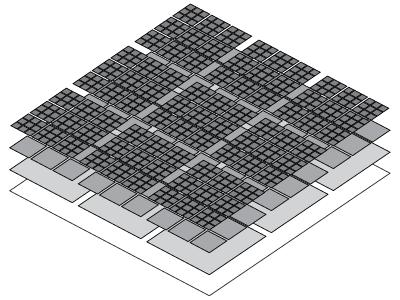# Fractal Sudoku

#### Algorithm Translation, Implementation, and Graphical Improvements

I enjoy Sudoku puzzles as diversions. I found a blog post by Peter Norvig where he describes a Sudoku Solver he wrote in Python. I translated his algorithm to ActionScript 3, and built a program that drills down into a series of unique but related Sudoku puzzles.

## Visit ProjectA Sudoku puzzle is a 9 by 9 grid, subdivided into nine 3 by 3 regions (these regions are labeled in the image with large grey numbers). The puzzle is considered solved when each row, column, and region contains the numerals 1 through 9 exactly once.

## Each puzzle has 9 regions.

My Fractal Sudoku is really just a proof-of-concept, considering that all of the puzzles are already solved by the program. The user is able to navigate through these solved puzzles.This image shows the original puzzle in the upper-left corner. If the user were to click in the first region of this puzzle, a new puzzle would be created. Each 3 by 3 region of the new puzzle is related to the clicked region of the original puzzle.

## Clicking spawns a related puzzle.

Notice that the upper left square of each 3 by 3 region has been seeded with the number from the corresponding square of the original puzzle.Every click progresses one level deeper into the puzzles. At level zero, there is only 1 puzzle. This level zero puzzle has 9 regions, so at level one there are 9 more puzzles.

## Each level n adds 9n more puzzles.

Each of the 9 level one puzzles has its own 9 regions to expand, so at level two there are 81 more puzzles. Each of level two's 81 puzzles has 9 regions to expand, so at level three there are 729 more puzzles. It quickly becomes overwhelming; in these first four levels there are 1 + 9 + 81 + 729 = 820 puzzles!